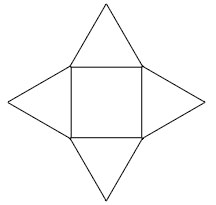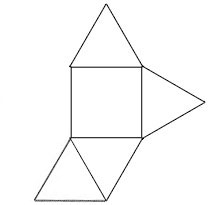SEARCH HOMEMath Central Quandaries & QueriesMiss, a teacher: How many different nets does a square-based pyramid have?Hi Miss,

The number of "nets" of what a solid shape would look like if we "unfolded it. A square pyramid would have a square and four triangles. For example:This is the most obvious example of a net of a square based pyramid. Here is a less obvious example of a net:How many more times could you do this before you begin to repeat the nets?

Hope this helps,

JaniceMath Central is supported by the University of Regina and The Pacific Institute for the Mathematical Sciences.